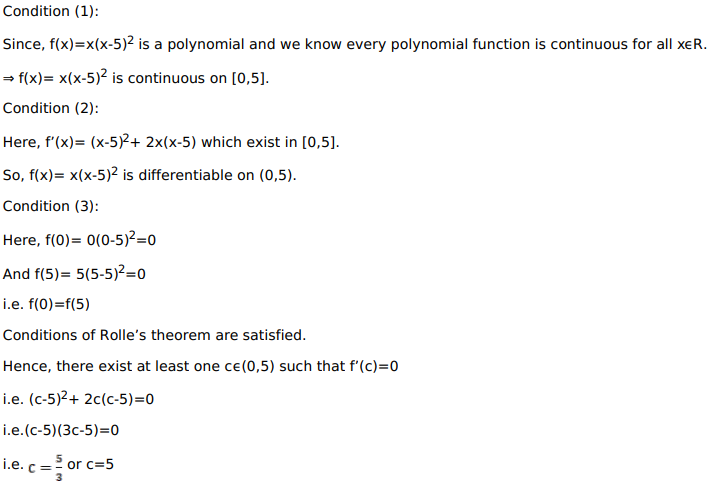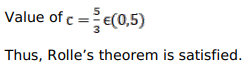# Verify Rolle's theorem for each of the following functions:

Question:

Verify Rolle's theorem for each of the following functions:

Show that $f(\mathrm{x})=\mathrm{x}(\mathrm{x}-5)^{2}$ satisfies Rolle's theorem on $[0,5]$ and that the value of $c$ is $(5 / 3)$

Solution: